﻿ A Special Case of Symmetric Matrices and Their ApplicationsAmerican Journal of Computational and Applied Mathematics

p-ISSN: 2165-8935    e-ISSN: 2165-8943

2019;  9(4): 110-118

doi:10.5923/j.ajcam.20190904.03### A Special Case of Symmetric Matrices and Their Applications

Ognyan Ivanov Zhelezov

Part-time lecturer, Nikola Vaptsarov Naval Academy, Varna, Bulgaria

Correspondence to: Ognyan Ivanov Zhelezov, Part-time lecturer, Nikola Vaptsarov Naval Academy, Varna, Bulgaria.
 Email: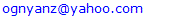Abstract

This article presents a special case of symmetric matrices, matrices of transpositions (Tr matrices) that are created from the elements of given n-dimensional vector X∈Rn, n=2m, m∈N. Has been proposed algorithm for obtaining matrices of transpositions with mutually orthogonal rows (Trs matrices) of dimensions 2, 4, and 8 as Hadamard product of Tr matrix and matrix of Hadamard and has been investigated their application for QR decomposition and n-dimensional rotation matrix generation. Tests and analysis of the algorithm show that obtaining an orthogonal Trs matrix of sizes 4 and 8 that rotates a given vector to the direction of one of the coordinate axes requires less processing time than obtaining a Housholder matrix of the same size.

Keywords: Mathematics of computing, Mathematical analysis, Numerical analysis, Computations on matrices

Cite this paper: Ognyan Ivanov Zhelezov, A Special Case of Symmetric Matrices and Their Applications, American Journal of Computational and Applied Mathematics , Vol. 9 No. 4, 2019, pp. 110-118. doi: 10.5923/j.ajcam.20190904.03.

### 1. Introduction

This article presents a special case of symmetric matrices, matrices of transpositions (Tr matrices) that are created from the elements of given n-dimensional vector X∈Rn, n=2m, m∈N, where value of every matrix’s element is equal to this element of vector X, which index is equal to bitwise XOR of zero based row and column indices of matrix’s element. It has been proved that elements of every two rows makes n/2 fours of elements, in which elements, having different row and column indices (we name them “diagonal elements”) consists the value of the same element of the given vector X. It has been defined type of matrices of transpositions (Trs matrices) having are mutually orthogonal rows, as Hadamard product of Tr matrix and n-dimensional Hadamard matrix having defined ordering of rows against Sylvester-Hadamard matrix. Has been investigated the application of Trs matrix for QR decomposition and n-dimensional rotation matrix generation.

### 2. Definition of Tr Matrix & Notations

Matrix of Transpositions (Tr matrix) is square matrix with dimension n=2m, m∈N, which rows are permutations of elements Xk, k∈[0, n-1] of given n-dimensional vector X and the values of elements Trp,q of matrix are obtained from the elements of given vector as follows: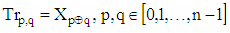(1)
where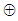denotes operation „bitwise exclusive or“ (XOR) , .
When it is needed to denote from which vector have been obtained the values of Tr matrix’s elements, we will use for the Tr matrix denotation Tr(X), and for its elements - denotation Tr(X)p,q.
As it given in (1), we will use zero based indices for vector’s elements and for Tr matrix’s rows and columns: p,q = 0,1,…,n-1.
As it can be seen from the definition of the matrix, it contains only the values of the elements of a given vector. What sets it apart from other similar matrices is the “property of the fours”, which is described below
The figure, given below shows 8-dimensional Tr matrix, created from arbitrary vector X=(x0,x1, … x7)T.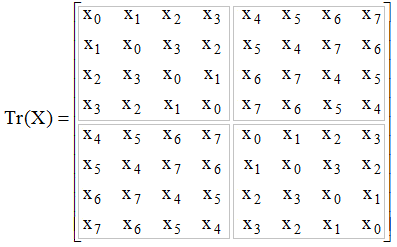Figure 1. 8-dimensional Tr matrix created from vector X=(x0,x1, … x7)T
The example, given below, consists code of Matlab function, which creates and returns Tr matrix obtained from n-dimensional vector X where n=2m, m∈N.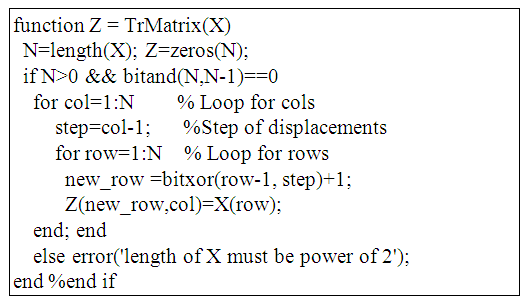Example 1. Matlab function, which creates and returns Tr matrix obtained from given n-dimensional vector X, n=2m, m∈N

### 3. Properties of Tr matrices

Properties of Tr matrices in most of cases are determined by the properties of bitwise XOR operation which relates indices of given vector X and indices of matrix’s elements, as it is given in (1). Below are listed and proved some of them, which we consider as most fundamental.

#### 3.1. Tr matrix is symmetric matrix

This property follows from commutativity of bitwise XOR operation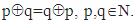From definition of Tr matrix we have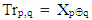and taking in consideration commutativity of XOR operation we have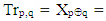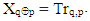which proves that Tr matrix is symmetric.

#### 3.2. Every One Row and Column of Tr Matrix Consists all n Elements of Given Vector X without Repetition

Since Tr matrix’s rows and columns and given vector X have the same dimension, it is enough to prove that every row and column consists elements of given vector X without repetition. To prove that every row of Tr matrix consists elements of given vector X without repetition we will prove that if two elements of the same row Trp,q и Trp,r consists value of the same element of the given vector X, then indices of their columns are equal, i.e. q=r which means that Trp,q and Trp,r denotes the same element of Tr matrix.
Proof: Let's examine two arbitrary elements Trp,q and Trp,u of Tr matrix’s row p. Since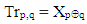and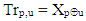(1) and according to condition above we have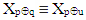which mean thatMultiplying two sides of this equation by p, according properties of XOR operation, we get: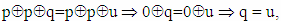(2)
which shows, that only one element of Tr matrix’s row can consists selected element of given vector X, so can't exists repetitions of the vector’s elements in Tr matrix’s rows. Since Tr is symmetric matrix, the same is valid for matrix’s columns Q.E.D.

#### 3.3. First Row & First Column of Tr Matrix Consists Elements of Given Vector X in Normal Order (without Inversions)

This property is determined by the properties of bitwise XOR operation too. If one element Trp,q of Tr matrix belongs to the first row (i.e. p=0), then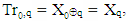which means that values of elements of first row are equal to the values of elements of given vector X having the same indices, i.e. in normal order. Since Tr is symmetric matrix, the same is valid for first matrix’s column Q.E.D.

#### 3.4. Property of the Fours

This property, named “property of the fours” presents relation between four Tr matrix’s elements. It is defined as follows: If two elements of different Tr matrix’s rows are equal to the same element of the given vector X, then the exchange of their column indices gives indices of another two Tr matrix’s elements, that are equal also to the same element of the given vector X (but different from this for the first two). On the scheme of Tr matrix (Figure 2) the fours of elements are located at the vertices of rectangles, such as the diagonal elements of every rectangle contain the same element of the vector X.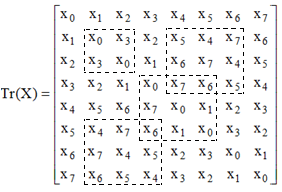Figure 2. Example of fours of elements in 8-dimensional Tr matrix, for which it is satisfied Tr-property
Let's look at the elements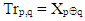and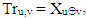for the indices of which is satisfied equality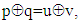that is they contain the same element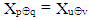of vector X. We will prove that from this follows that for the elements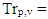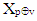and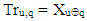is satisfied equality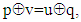that is they contain the same element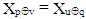of given vector X too.
Proof: Let's look at the equation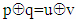which is satisfied on condition. Multiplying by p to the right of the two sides of the equation, we get: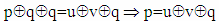(3)
whence by multiplying the two sides of equality by v and using the commutativity of XOR operation, we get:(4)
which means that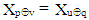so Trp,v and Tru,q consists the same element of given vector X which proves the property.
This property, that we shall hereafter call “Tr-property” is specific to Tr matrix. The figure above shows an example of 8-dimensional Tr matrix in which are indicated by dotted lines several groups of 4 elements, which form rectangles with equal diagonal elements (which mean, that they satisfy Tr-property).

#### 3.5. Every Two Rows of Tr Matrix Consists n/2 Fours of Elements with the Same Values of the Diagonal Elements

Let's look at two arbitrary elements Trp,q and Tru,q from the same column q of two different Tr matrix’s rows p and u, p≠u. According to Tr-property, for those two elements in Tr matrix are contained two corresponding elements Trp,v and Tru,v, for which are satisfied equations Trp,q = Tru,v и Trp,v = Tru,q. Since in each fours of elements two elements are contained in one of the rows and two in the other, to prove that the number of fours is n / 2, it is sufficient to prove that each element of row p is involved in only one fours with elements of row u.
Proof: Suppose the element Trp,q of p participates in two fours with elements of row u. This would mean that there are two elements Tru,v and Tru,s in the row u of the Tr matrix, for indices of which are satisfied the equations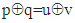and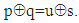But it would follow, that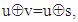where by multiplying the two sides of equality by u we get v=s, which mean that elements are the same, and therefore the element Trp,q participates in only one fours with elements from row u. Since every one of fours consists two elements of row p, it follows that rows p and u consists n/2 fours of elements, Q.E.D.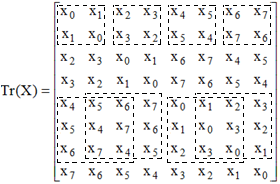Figure 3. Fours of elements in 8-dimensional Tr matrix between elements of rows 1-2 and 5-7, for which it is satisfied Tr-property
The figure above shows fours of elements between first-second and fifth-sixth rows of 8-dimensional Tr matrix shown in Figure 1. Given that the elements of each row of the Tr matrix participate in n/2 fours of elements with elements from each of the other rows, it is easy to calculate the number of the fours of elements in Tr matrix, for which Tr-property is satisfied. Given that the elements of the k-th row participate in n / 2 fours with elements from the remaining k-1 rows with a smaller indices, for the total number of the fours Nfours in n-dimensional Tr matrix we get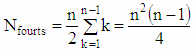(5)

### 4. Matrix of Transpositions with Mutually Orthogonal Rows

Matrix of Transpositions with mutually orthogonal rows (Trs matrix) is square matrix with dimension n=2,4 or 8, which is obtained as Hadamard product, (denoted by ○) of Tr matrix and n-dimensional Hadamard matrix whose rows (except the first one) are rearranged relative to the rows of Sylvester-Hadamard matrix in order R=[1, r2, … rn]T, r2, … rn ∈[2,n] for which the rows of the resulting Trs matrix are mutually orthogonal.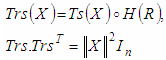(6)
where: ○ denotes operation Hadamard product,
In is n-dimensional Identity matrix
H(R) is n-dimensional Hadamard matrix, which rows are interchanged against the Sylvester-Hadamard matrix in given order R=[1, r2, … rn]T, r2, … rn ∈[2,n] for which the rows of the resulting Trs matrix are mutually orthogonal.
X is the vector from which the elements of Tr matrix are derived.
It is important to note, that the ordering R of Hadamard matrix’s rows (against the Sylvester-Hadamard matrix) does not depend on the vector X but only on its size.
The figure below shows 8-bit Trs matrix for a vector X=[x0,x1, … x7]T and for H(R) rows ordering R=[1 2 8 3 6 7 4 5]T.
Since, by definition, the first row of the H(R) matrix contains the first row of the n-dimensional Sylvester-Hadamard matrix, and all elements of the first column of the Sylvester-Hadamard matrix are +1, it follows that the first row and the first column of the Trs matrix contain the components of the vector X without inversions, as can be seen in the figure below.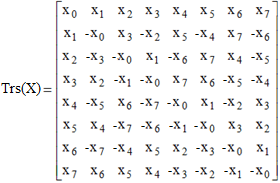Figure 4. Schema of 8-dimensional Trs matrix for vector X=[x0,x1, … x7]T
The following example shows the code of a Matlab function that creates and returns a Trs matrix for a given vector X of size n = 2, 4, or 8.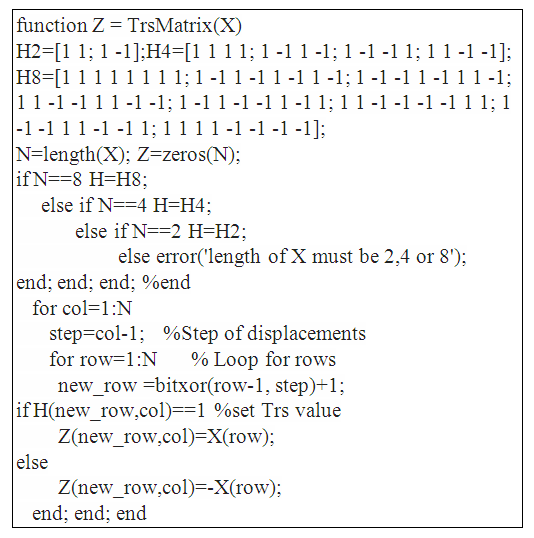Example 2. Matlab function, that creates and returns a Trs matrix for a given vector X of size n = 2, 4, or 8

### 5. Fours of Elements in the Trs и H(R) Matrices

We shall hereafter call Trs fours of elements (or just Trs-fours) the elements (Trsp,q, Trsp,v, Trsu,q, Trsu,v) of rows p and u of Trs matrix, where p,q,u,v∈[0,n-1] and p≠u, if their corresponding elements from the Tr matrix (Trp,q, Trp,v, Tru,q, Tru,v) satisfy the Tr-property. As proved in 3.3, to have this, it is necessary for the indices p, q, u, v of the elements to satisfy the equality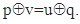Similarly, we will call H fours of elements (or just H-fours) elements H(R)p,q, H(R)p,v, H(R)u,q, H(R)u,v of rows p and u of H(R) matrix, where p,q,u,v∈[0,n-1] and p≠u, if their corresponding elements from the Tr matrix (Trp,q, Trp,v, Tru,q, Tru,v) satisfy the Tr-property.

### 6. Properties of Trs Matrices

The properties of the Trs matrix are determined by its definition and by the method of its preparation, given in (5).

#### 6.1. The Fours of Elements in the Trs Matrix Form Pairs of Orthogonal Two-Dimensional Vectors – Trs-Property

The set vector X uniquely defines the Tr matrix, while for the n-dimensional Hadamard matrix H(R)n the definition only requires that the rows of the matrix have to be interchanged relative to the Sylvester-Hadamard matrix for ordering R=[1, r2, … rn]T, so that the rows of the resulting Trs matrix are mutually orthogonal. This mutual orthogonality of the rows of the Trs matrix is obtained when the two-dimensional vectors in the Trs-fours are mutually orthogonal. This property of the Trs-fours (orthogonal pairs of two-dimensional vectors) hereafter we shall call Trs-property. To demonstrate this property, let's look at two rows of the 8-bit Tr matrix from Figure 1 (such as rows 1 and 2) and the result of their Hadamard product by rows from the Hadamard matrix. Rows 1 and 2 of the 8-bit Tr matrix have the following contents: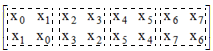Figure 5. Schema of the fours of elements in the first two rows of an 8-bit Tr matrix
where dotted lines indicates the fours of elements that satisfy Tr-property. If these rows are multiplied as Hadamard product by the first two rows of the Sylvester-Hadamard matrix.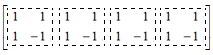Figure 6. Schema of the first two rows of Silvester-Hadamard matrix
The following first two rows of the Trs matrix are obtained: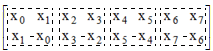Figure 7. Schema of the fours of elements in the first two rows of an 8-dimensional Trs matrix
As can be seen from the diagram, the Trs-fours form groups of mutually orthogonal two-dimensional vectors and, as a result, the first two rows of the Trs matrix are orthogonal. Similarly, if the corresponding two-dimensional vectors of the Trs-fours of any two rows in the Trs matrix are mutually orthogonal, then the entire rows will be mutually orthogonal.

#### 6.2. The Fours of Elements in the Hadamard H(R) n-dimensional Matrix must Form Pairs of Orthogonal Two-Dimensional Vectors

We will prove that in order to obtain the orthogonality of the Trs-fours in the Trs matrix (which also implies the mutual orthogonality of the rows), it is sufficient the corresponding H-fours in the n-dimensional matrix of Hadamard H(R)n to contain orthogonal vectors.
Let's look at the Trs-fours (Trsp,q, Trsp,v, Trsu,q, Trsu,v), p,q,u,v∈[0,n-1] of rows p and u of Trs matrix. According to the definition of the Trs matrix, they are obtained by element by element multiplication (Hadamard product) of the corresponding elements of the Tr matrix (Trp,q, Trp,v, Tru,q, Tru,v), which satisfy T-property and corresponding elements (H(R)p,q, H(R)p,v, H(R)u,q, H(R)u,v of Hadamard matrix H(R). To be two-dimensional vectors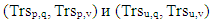(7)
as mutually orthogonal, their scalar product must be zero: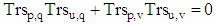(8)
whence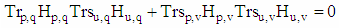(9)
whence, given that (according to the Tr-property) Trp,q = Tru,v and Trsp,v = Trsu,q it follows that in order for the two-dimensional vectors (6) to be orthogonal to each other, for the corresponding four elements of the Hadamard H (R) matrix have to be satisfied the equality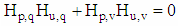(10)
from which follows that vectors (Hp,q, Hp,v) и (Hu,q, Hu,v) have to be orthogonal too, Q.E.D.

#### 6.3. The Trs Matrix Created by a Unit Vector X is an Orthogonal Matrix

This property follows from the definition of the Trs matrix (4), according to which the rows of the Trs matrix are mutually orthogonal and contain transpositions of the elements of the given vector X. Then for an n-dimensional Trs(X) matrix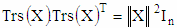(11)
where In is n-dimensional identity matrix. If X is unit vector then ||X||=1 and Trs(X).Trs(X)T=In and consequently Trs(X) is an orthogonal matrix.

#### 6.4. The Trs Matrix has Symmetrical Connectivity of the Elements of the First Row and Column and Antisymmetric Connectivity of the Other Elements Except the Diagonal Elements

As indicated in (6) the first row and the first column of the Trs matrix contains the elements of the given vector X without inversions. We will prove that from the mutual orthogonality of the rows of Trs the matrix follows that the elements which are not contained in the first row and the first column are antisymmetric (skew-symmetric).
We look at the Trs-fours (Trs0,0, Trs0,u, Trsu,0, Trsu,u), which contain the first diagonal element Trs0,0, element from the first row Trs0,u, element of the first column Trsu,0 and diagonal element Trsu,u. As Trs0,0=X0, Trs0,u=Xu, Tru,0=Xu then according Tr-property follows that diagonal element Tru,u = -X0, therefore diagonal elements of Trs the matrix except for Tr0,0 contain the first element of the given vector X with a negative sign Tru,u = -X0, u∈[1,n-1].
Let’s now look at the Trs-fours of elements (Trsu,u, Trsu,v, Trsv,u, Trsv,v), u,v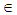[1,n-1]., u≠v, which contain two diagonal elements Trsu,u and Trsv,v and two diagonally spaced elements Trsu,v and Trsu,v. As (shown above) Trsu,u = -X0.и Trsv,v = -X0, then according Trs-рproperty it follows that the diagonally spaced elements Trsu,v и Trsv,v have opposite signs Trsu,v = -Trsv,u Q.E.D.

#### 6.5. The Trs Matrix Created from Unit Vector X is Matrix of Reflection

Let’s have transpositions matrix with mutually orthogonal rows Trs(X), obtained from an arbitrary n-dimensional unit vector X=[x0,x1, … xn-1]T, ||X||=1, n∈[2,4,8].
As shown in property 6.3, matrix Trs(X), obtained from unit vector is an orthogonal matrix, therefore ||det(Trs(X))||=1. We will prove that det(Trs(X))=-1, which will mean that Trs matrix is matrix of reflection.
Proof:
As proved in 6.4, Trs matrix has symmetric dependence of the elements of the first row and column and antisymmetric dependence of the other elements. Then the matrix obtained as a product of Trs(X) and a diagonal matrix D in which d1,1=-1 and di,i=1 for i≠1 can be represented as the sum of an skew-symmetric matrix S and matrix x0In, where In is an n-dimensional identity matrix as follows: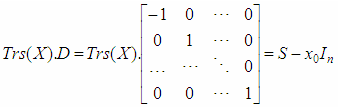(13)
In example for n = 4 and the transposition of the rows of H(R) R = [1,2,4,3]T the matrix equation (13) will look as follows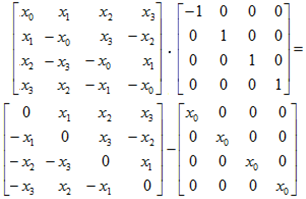Figure 8. Representing the product of Trs (X) and matrix of a single inversion as difference of a symmetric and diagonal matrix
Hence, given that D is a diagonal matrix and therefore D-1=D, we get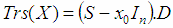(14)
whence, considering that det(D)=-1 for the determinant of Trs matrix is obtained
As is known , , , ,  the determinant of real skew-symmetric matrices is nonnegative det(S)≥0, and their eigenvalues are either zero or purely imaginary. Thus, the n-bit skew-symmetric matrix S has n/2 pairs of zeroed or imaginary eigenvalues λk=±iβk, k=1,2,…n/2, βk∈R. These eigenvalues are solutions of the characteristic equation det(S-λI)=0 of an skew-symmetric matrix S. Similarly, the eigenvalues γk of the matrix S-x0I are solutions of the characteristic equation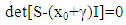(15)
If in (15) we put x0+γ=λ we obtain the characteristic equation of the skew-symmetric matrix S, therefore, the pairs of complex conjugate eigenvalues γk of matrix S-x0I can be obtained from the eigenvalues of the matrix S as follows: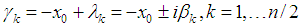(16)
Hence for determinant of matrix S - x0In we get: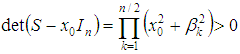(17)
whence after substitution in (14) is obtained det(Trs(X))<0. Then from property 6.3 it follows that for every unit vector X∈Rn, ||X||=1 is satisfied det(Trs(X))=-1 and therefore Trs(X) is matrix of reflection Q.E.D.

### 7. Obtaining n-dimensional Hadamard Matrix H(R)n for which the Rows of the Trs Matrix are Mutually Orthogonal

As shown in 6.1. to obtain from (5) a Trs matrix whose rows are mutually orthogonal, it is enough the two-dimensional subvectors in the fours of elements in n-dimensional Hadamard matrix H(R)n to be mutually orthogonal (9). Given that the elements of Hadamard matrix are either +1 or −1, from (10) we get: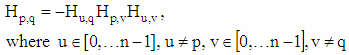(12)
Each element of the k-th row of H(R)n matrix, k∈[1,…n-1] is the first element in k fours of elements. To be the pairs of subvectors in each of these fours as mutually orthogonal, its value must be equal to the product of the other elements of the fours, taken with a negative sign. Thus, the value of each element of the k-th row must satisfy k equations (12), and in total for all elements the number of equations is given by formula (5). Moreover, the values of the elements on the right-hand side of equations (12) are not independent, and each of them must satisfy k equations (12), where k is the number of the row in which the element is located.
For practical application of the Trs matrix it is necessary to determine for which values of n, i.e. for which dimensions of the Tr matrix exists n-dimensional Hadamard matrices H(R)n, for which the two-dimensional vectors in all fours of elements are mutually orthogonal. This in practice means to determine whether for a given value of n exists orderings R=[r1,r2,…rn]T of Sylvester-Hadamard matrix rows, for which the two-dimensional vectors in all fours of elements are mutually orthogonal.
In this article, we do not offer an analytical solution to this problem, nor proof that an analytical solution cannot be obtained. We have used the “brute force” method to determine for which values of n exists Trs matrices with mutually orthogonal rows. Have been tested all possible orderings of Sylvester-Hadamard matrix rows for vector dimensions of n =2m, m∈N to find all arrangements, for which the rows of Trs matrix are mutual orthogonal.
A Matlab test program was created to obtain for a given size n all Hadamard matrices (which mean all orderings R=[r1,r2,…rn]T of Sylvester-Hadamard matrix), for which the rows of the Trs matrices are mutually orthogonal. Tests show that Hadamard matrices H(R)n, for which two-dimensional vectors in all fours of elements are mutually orthogonal, exists for n=2, 4 and 8 but do not exist for n≥16. Since Trs matrices are obtained as Hadamard product of Tr matrix and H(R)n matrix, this means that Trs matrices whose rows are mutually orthogonal exist only for dimensions n =2, 4 and 8.

### 8. Practical Application of Trs Matrices

By definition, Trs(X) is a matrix whose rows are mutually orthogonal and contain transpositions of a given vector X, while the first row contains the values of X without inversions. Therefore, if ||X||=1 then the multiplication of Trs(X/||X||) by X gives unit vector to the direction of the coordinate axis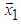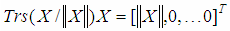(18)
The rotation of a given vector X to the direction of the coordinate axis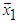is base operation of the NRMG algorithm for obtaining an n-dimensional rotation matrix  and of algorithm for QR decomposition of a matrix , . Base operation of those two algorithms is rotation (or reflection) of given n-dimensional vector to the direction of one of coordinate axes. For “dense” matrices as the most effective method is considered Householder reflection , , , while for “sparse” matrices more effective could be the method using Givens rotations.
The use of Trs matrices is an alternative method, which in some cases may be more effective than both Householder reflections and Givens rotations. Tests and analysis of the algorithm show that obtaining an orthogonal Trs matrix of sizes 4 and 8 that rotates a given vector to the direction of one of the coordinate axes requires less processing time than obtaining such Housholder matrix. A description of the testing is given in "Numerical Experiments".
Rotation of n-dimensional vector to the direction of the coordinate axis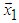using Trs matrices.
As is known , , , any positive integer n∈N, n<2m+1 can be represented in a binary number system as a polynomial in terms of 2 by coefficients ak=[0,1] as follows: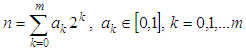(19)
whence the aggregates collected for k≥3 we get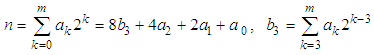(20)
Formula (20) shows how a given n-dimensional vector X can be represented as the sum of vectors with eight, four, two and one non-zero coordinates, respectively. For example, if we have vector X=[x6,x5,x4,x3,x2,x1]T it can be represented a sum of two vectors, which have four and two non-zero elements respectively: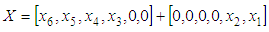(21)
The Trs matrices obtained from these vectors can be used to perform basic operations for reflection of 8, 4 and 2-dimensional vectors to the direction of one of coordinate axes, for example to the direction of the axis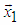. The figure below shows graphically the transformation operations for 2, 4 and 8-dimensional vectors through Trs matrices. The unit for rotating a two-dimensional vector through a Trs matrix is equivalent to multiplying with Givens matrix for rotating a 2-dimensional vector in coordinate plane (xk, xk+1), but Trs matrix is matrix of reflection, while Givens matrix is matrix of rotation.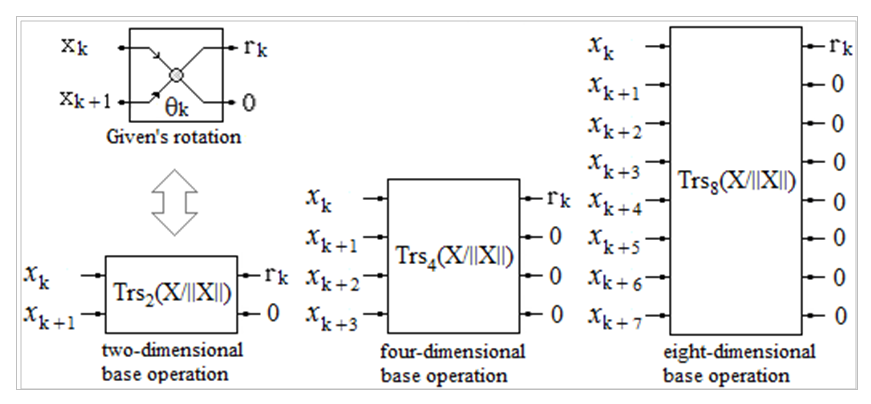Figure 9. Schema for transformation of 2, 4 and 8-dimensional vectors using Trs matrices
For example, the rotation of a 10-dimensional vector X to the direction of the coordinate axis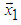by means of Trs matrices can be represented by the following block diagram (Figure 10). From the first eight elements of the given 10-dimensional vector X, is obtained matrix Trs8(X8/||X8||), which reflects the 8- dimensional vector X8 to the direction of the axis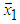. From a two-dimensional vector X2=[x9,x10]T is obtained matrix Trs2(X2/||X2||), which reflects X2 to the direction of the axis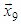. In the second stage of the algorithm through matrix Trs2(X22/||X22||) the vector X22=[r8,r2]T is reflected in the coordinate plane (x1, x9) which gives 10-dimensional output vector Y=[r10,0,...,0]T. Since Trs are orthogonal matrices, then ||Y||=[r10,0,...,0]T =||X|| and consequently |r10|=||X||.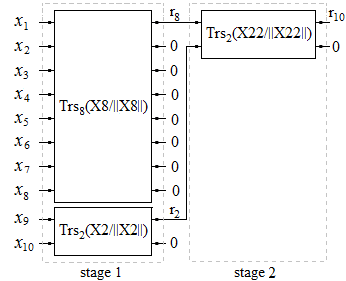Figure 10. Schema for rotation of 10-dimensional vector to the direction of axis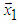using Trs matrices
It is important to note that all matrices of stages Si are n-dimensional block matrices in which the blocks are Trs matrices for base operations (Figure 9) or single diagonal elements. For example, to reflect a 10-dimensional vector X the matrices of stages will have the following form: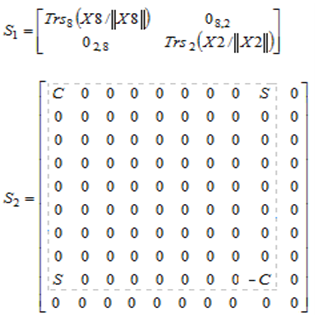(21)
where### 9. Numerical Experiments

Has been created Matlab program to test the application of Trs matrices for QR decomposition , . The following example gives the code of a program that performs QR decomposition of a matrix obtained with a random number generator using Trs matrices or Householder transformation.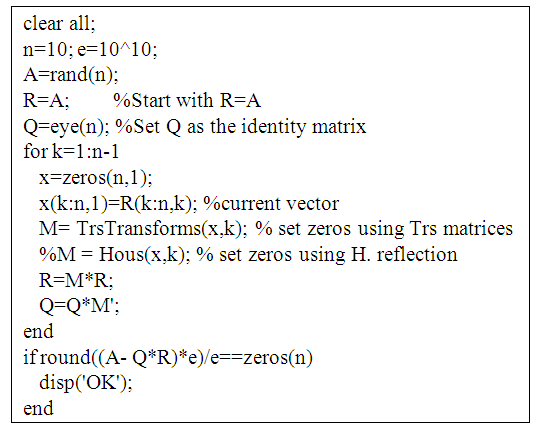Example 3. Code of Matlab for QR decomposition using Trs matrices or Householder reflection
In the example, for each iteration of Matlab for loop, the function TrsTransforms(x, k) produces an orthogonal matrix M, which, when multiplied by the current matrix R, produces a matrix R with zero values of the elements of the k-th column with an index greater than k. TrsTransforms (x, k) receives matrix M as a product of matrices, each containing a block Trs matrices (Figure 9) and diagonal elements. The Matlab code of TrsTransforms (x, k) is given as Example 2.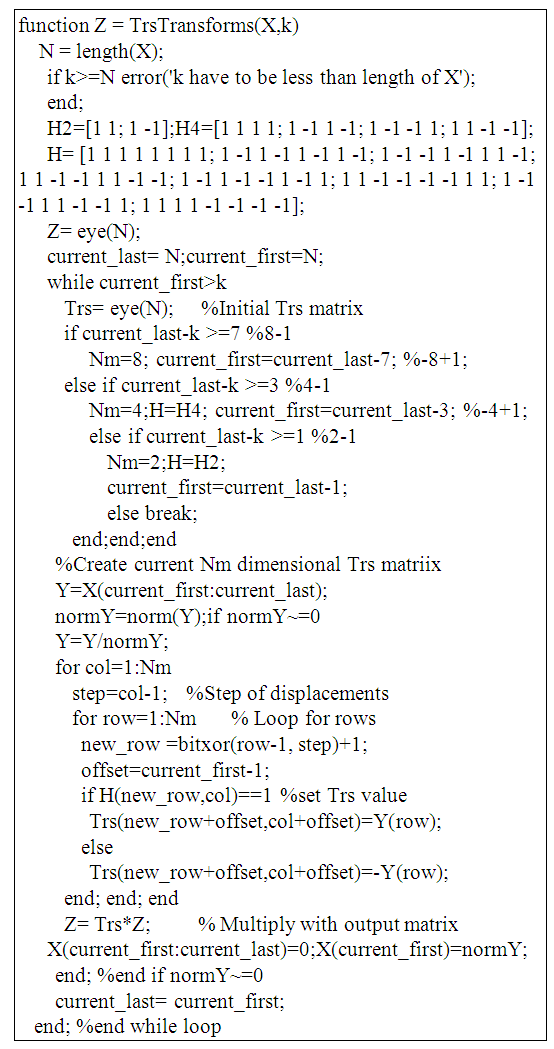Example 4. Code of Matlab function, which returns orthogonal matrix M, that zeroes elements of k-th column under k-th index of R matrix using Trs block matrices
The same result can be obtained if, in Example 3, instead of function TrsTransforms(x, k), we use function Hous(x, k), which obtains the matrix M through Householder transformation . Just remove command M= TrsTransforms(x,k); and the comment symbol "%" at the beginning of the line %M = Householder(x,k);. The following example shows the Matlab code of the function Householder(x,k).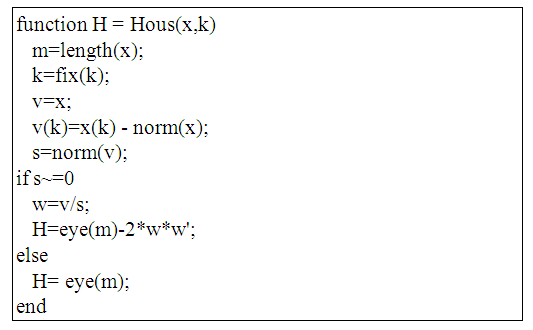Example 5. Code of Matlab function, which returns orthogonal matrix M, that zeroes elements of k-th column under k-th index of R matrix using Householder transformation

### 10. Algorithmic Efficiency

As is known , , , , , the Householder transformation is considered to be the most efficient and stable method for the QR decomposition of “deep” matrices.
When obtaining a Householder matrix using the function Hous(x, k) for a given n-dimensional vector x, two normalizations of k-bit vectors and k2 multiplications is performed.
When calculating a Trs matrix for vector X∈Rn where n = 4 or n = 8, using the function TrsMatrix(X), it calculates one normalizations of the given vector x and executes two nested cycles in which are executed n2 bitwise XOR operations.
For comparison, when calculating a Householder matrix for vector X∈Rn using the Hous(X,1) function, it calculates two normalizations and performs two nested cycles in which 2n2 multiplications are performed.
A C ++ test program was created1 to compare the execution time of functions to obtain an 8-bit Trs matrix and an 8-bit Householder matrix. The results of execution of C ++ test program shows that for the 4 and 8 dimensional vectors, the calculation of the Trs matrix requires less processing time than the calculation of the Householder matrix.
However, when testing QR decomposition, the results show that for “dense” matrices, using the TrsTransforms function (which uses Trs matrices) instead of Hous(x,k) function (which uses Householder matrices) requires several times more execution time.

### 11. Conclusions

In this article, we presents a special case of symmetric matrices, matrices of transpositions (Tr matrices) that are constructed from the elements of a given n-dimensional vector X∈Rn, n=2m, m∈N. An algorithm for obtaining matrices of transpositions with mutually orthogonal rows (Trs matrices) of dimensions 2, 4, and 8 as Hadamard product from the symmetric Tr matrix and Hadamard matrix is proposed and the possibility of using them for QR decompozition and obtaining a rotation matrix according to the NRMG algorithm is considered.

### Note

1. https://www.researchgate.net/publication/335570264_Test_of_Trs_and_Housholder_Matrices

### References

  O. I. Zhelezov, 2017, N-dimensional Rotation Matrix Generation Algorithm, American Journal of Computational and Applied Mathematics, Vol. 7 No. 2, 2017, pp. 51-57. doi: 10.5923/j.ajcam. 20170702.04.  G. A. Korn, T. M. Korn, 1961, Mathematical Handbook for Scientists and Engineers (1st ed.), New York: McGraw-Hill. pp. 55–79.  G. Strang, 2006, Linear Algebra and its Applications, Thomson Learning Ink, pages 69-135, ISBN 0-03-010567.  D. A. Harville, 1997, Matrix Algebra from Statistician’s Perspective, Softcover.  B. Buchberger, 1985, Multidimensional Systems Theory - Progress Directions and Open Problems in Multidimensional Systems, Reidel Publishing Company.  G. H. Golub, C. F. Van Loan, 1996, Matrix Computations, 4rd edition. Johns Horkins University Press, Baltimore.  M. Cosnard, Y. Robert. Complexity of parallel QR factorization. J. ACM 33, 4 (August 1986), 712-723. DOI=10.1145/6490.214102, 1986.  A. H. Sameh, D. J. Kuck. On Stable Parallel Linear System Solvers. J. ACM 25, 1 (January 1978), 81-91. DOI= http://dx.doi.org/10.1145/322047.322054, 1978.  N. J. Higham, 1996, Accuracy and Stability of Numerical Algorithms, SIAM, Philadelfia.  G. W.Steward, 1976, The economical storage of plane rotations, Numer. Math, 25, 2 1976, 137-139.  Matlock, H., and Reese, L.C., 1960, Generalized solutions for laterally loaded piles., Journal of Soil Mechanics and Foundation, 86(5), 63–91.  N. K. Bose, 1985, Multidimensional Systems Theory: Progress, Directions, and Open Problems. D. Reidel Publishing Co., Dordrecht, The Netherland.  A. S. Householder, 1958, “Unitary triangularization of a nonsimetric matrix”, J. ACM 5, 339-342, 1958.  E. Anderson, 2000, “Discontinuous Plane Rotations and the Symmetric Eigenvalue Problem” LAPACK Working Note 150, University of Tennessee, UT-CS-00-454, December 4, 2000. page 2.  Gentle, J. E. "QR Factorization." §3.2.2 in Numerical Linear Algebra for Applications in Statistics. Berlin: Springer-Verlag, pp. 95-97, 1998.  I. R. Shafarevich, A. Remizov, 2013, Linear Algebra and Geometry, Springer, pages 133-160, ISBN 978-3-642-30993-9.  S. R. Davis C++ for dummies. IDG Books Worldwide, 2000.  Hedayat, A., Wallis, W. D., Hadamard Matrices and their Applications, TheAnnals of Statistics, Vol. 6, Number 6, pp 1184-1238, 1978.  Wallis, W.D., Street, A. P., Wallis, J. S., Combinatorics: Room Squares, Sum-freeSets, Hadamard Matrices, Lecture Notes in Mathematics, Vol. 292, Springer-Verlag,New York, 1972.  Scott, N. R., Computer Number Systems and Arithmetic, Prentice Aall, Englewood Cliffs, N.J. 1985.  Victor P. Nelson, H. Troy Nagle, Bill D. Carroll, and J. David Irwin, Digital Logic Circuit Analysis and Design, Prentice Hall, Inc., 1995.  St. Roman, Advanced Linear Algebra, second ed., 2005 Springer-Verlag, New York. pages 59-85, ISBN: 978-1-4757-2180-5.  J. E. Gentle, 2007, Matrix Algebra: Theory, Computations, and Applications in Statistics, Springer, page 180, ISBN 978-0-387-70872-0.